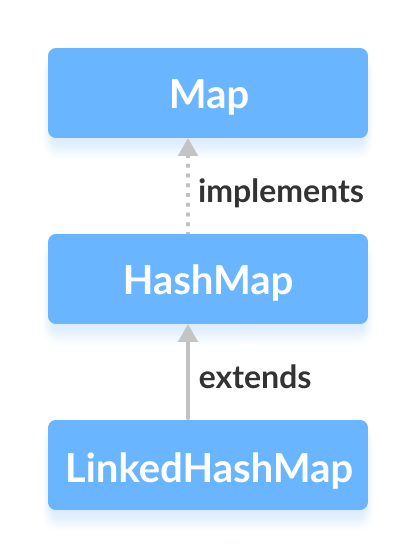In this tutorial, we will learn about the Java LinkedHashMap class and its operations with the help of examples.

The `LinkedHashMap` class of the Java collections framework provides the hash table and linked list implementation of the Map interface.

The `LinkedHashMap` interface extends the HashMap class to store its entries in a hash table. It internally maintains a doubly-linked list among all of its entries to order its entries.In order to create a linked hashmap, we must import the `java.util.LinkedHashMap` package first. Once we import the package, here is how we can create linked hashmaps in Java.

``````// LinkedHashMap with initial capacity 8 and load factor 0.6
``````

In the above code, we have created a linked hashmap named numbers.

Here,

• Key - a unique identifier used to associate each element (value) in a map
• Value - elements associated by the keys in a map

Notice the part `new LinkedHashMap<>(8, 0.6)`. Here, the first parameter is capacity and the second parameter is loadFactor.

• capacity - The capacity of this linked hashmap is 8. Meaning, it can store 8 entries.
• loadFactor - The load factor of this linked hashmap is 0.6. This means, whenever our hash map is filled by 60%, the entries are moved to a new hash table of double the size of the original hash table.

It's possible to create a linked hashmap without defining its capacity and load factor. For example,

``````//LinkedHashMap with default capacity and load factor
``````

By default,

• the capacity of the linked hashmap will be 16
• the load factor will be 0.75

Note: The `LinkedHashMap` class also allows us to define the order of its entries. For example

``````// LinkedHashMap with specified order
``````

Here, accessOrder is a boolean value. Its default value is `false`. In this case entries in the linked hashmap are ordered on the basis of their insertion order.

However, if `true` is passed as accessOrder, entries in the linked hashmap will be ordered from least-recently accessed to most-recently accessed.

## Creating LinkedHashMap from Other Maps

Here is how we can create a linked hashmap containing all the elements of other maps.

``````import java.util.LinkedHashMap;

class Main {
public static void main(String[] args) {
// Creating a LinkedHashMap of even numbers
evenNumbers.put("Two", 2);
evenNumbers.put("Four", 4);

numbers.put("Three", 3);
}
}
``````

Output

```LinkedHashMap1: {Two=2, Four=4}
```

The `LinkedHashMap` class provides methods that allow us to perform various operations on the map.

• `put()` - inserts the specified key/value mapping to the map
• `putAll()` - inserts all the entries from the specified map to this map
• `putIfAbsent()` - inserts the specified key/value mapping to the map if the specified key is not present in the map

For example,

``````

class Main {
public static void main(String[] args) {
// Creating LinkedHashMap of even numbers

// Using put()
evenNumbers.put("Two", 2);
evenNumbers.put("Four", 4);

// Using putIfAbsent()
evenNumbers.putIfAbsent("Six", 6);

numbers.put("One", 1);

// Using putAll()
numbers.putAll(evenNumbers);
}
}
``````

Output

```Original LinkedHashMap: {Two=2, Four=4}
New LinkedHashMap: {One=1, Two=2, Four=4, Six=6}
```

1. Using entrySet(), keySet() and values()

• `entrySet()` - returns a set of all the key/value mapping of the map
• `keySet()` - returns a set of all the keys of the map
• `values()` - returns a set of all the values of the map

For example,

``````import java.util.LinkedHashMap;

class Main {
public static void main(String[] args) {

numbers.put("One", 1);
numbers.put("Two", 2);
numbers.put("Three", 3);

// Using entrySet()
System.out.println("Key/Value mappings: " + numbers.entrySet());

// Using keySet()
System.out.println("Keys: " + numbers.keySet());

// Using values()
System.out.println("Values: " + numbers.values());
}
}
``````

Output

```LinkedHashMap: {One=1, Two=2, Three=3}
Key/Value mappings: [One=1, Two=2, Three=3]
Keys: [One, Two, Three]
Values: [1, 2, 3]
```

2. Using get() and getOrDefault()

• `get()` - Returns the value associated with the specified key. If the key is not found, it returns `null`.
• `getOrDefault()` - Returns the value associated with the specified key. If the key is not found, it returns the specified default value.

For example,

``````import java.util.LinkedHashMap;

class Main {
public static void main(String[] args) {

numbers.put("One", 1);
numbers.put("Two", 2);
numbers.put("Three", 3);

// Using get()
int value1 = numbers.get("Three");
System.out.println("Returned Number: " + value1);

// Using getOrDefault()
int value2 = numbers.getOrDefault("Five", 5);
System.out.println("Returned Number: " + value2);
}
}
``````

Output

```LinkedHashMap: {One=1, Two=2, Three=3}
Returned Number: 3
Returned Number: 5
```

• `remove(key)` - returns and removes the entry associated with the specified key from the map
• `remove(key, value)` - removes the entry from the map only if the specified key mapped to be the specified value and return a boolean value

For example,

``````import java.util.LinkedHashMap;

class Main {
public static void main(String[] args) {

numbers.put("One", 1);
numbers.put("Two", 2);
numbers.put("Three", 3);

// remove method with single parameter
int value = numbers.remove("Two");
System.out.println("Removed value: " + value);

// remove method with two parameters
boolean result = numbers.remove("Three", 3);
System.out.println("Is the entry Three removed? " + result);

}
}
``````

Output

```LinkedHashMap: {One=1, Two=2, Three=3}
Removed value: 2
Is the entry {Three=3} removed? True
```

Method Description
`clear()` removes all the entries from the map
`containsKey()` checks if the map contains the specified key and returns a boolean value
`containsValue()` checks if the map contains the specified value and returns a boolean value
`size()` returns the size of the map
`isEmpty()` checks if the map is empty and returns a boolean value

Both the `LinkedHashMap` and the `HashMap` implements the `Map` interface. However, there exist some differences between them.
• `LinkedHashMap` maintains a doubly-linked list internally. Due to this, it maintains the insertion order of its elements.
• The `LinkedHashMap` class requires more storage than `HashMap`. This is because `LinkedHashMap` maintains linked lists internally.
• The performance of `LinkedHashMap` is slower than `HashMap`.